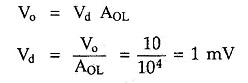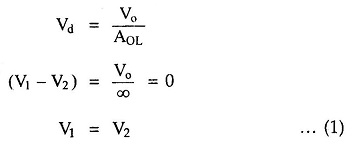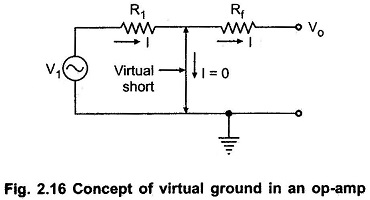## Op Amp Assumptions:

We can make two Op Amp Assumptions which are realistic and simplify the analysis of op-amp circuits to a great extent. The Op Amp Assumptions are useful and can be used to obtain the output expressions in variety of linear applications.

### Zero Input Current:

The current drawn by either of the input terminals (inverting and noninverting) is zero.

In practice, the current drawn by the input terminals is very small, of the order of μA or nA. Hence the Op Amp Assumptions of zero input current is realistic.

### Virtual Ground:

This means the differential input voltage Vd between the non inverting and inverting input terminals is essentially zero.

This is obvious because even if output voltage is few volts, due to large open loop gain of op-amp, the difference voltage Vd at the input terminals is almost zero.

e.g. if output voltage is 10 V and the AOL i.e. open loop gain is 104 thenHence Vd is very small. As AOL → ∞, the difference voltage Vd → 0 and realistically assumed to be zero for analyzing the circuits.Thus we can say that under linear range of operation there is virtually short circuit between the two input terminals, in the sense that their voltages are same. No current flows from the input terminals to the ground. The Fig. 2.16 shows the concept of the virtual ground. The thick line indicates the virtual short circuit between the input terminals.Now if the non-inverting terminal is grounded, by the concept of virtual short, the inverting terminal is also at ground potential, though there is no physical connection between the inverting terminal and the ground. This is the principle of virtual ground.

Thus from the equation (1), the voltage at the one input terminal of an op-amp can be realistically assumed to be equal to the voltage at the other input terminal.

This Op Amp Assumptions concept is very much useful in the analysis of closed loop op-amp circuits.

Scroll to Top